# Isolated singular point

for an element of an analytic functionA pointin the complex-plane satisfying the following properties: 1) the element ofdoes not have an analytic continuation along any path to; and 2) there exists a numbersuch that analytic continuation ofis possible along any path in the punctured neighbourhood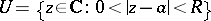of.

If a new element is obtained whenis continued analytically along a closed path inencircling, for example along the circle,, thenis called a branch point, or an isolated singular point of multi-valued character. Otherwise the element ofdefines a single-valued analytic function inandis called an isolated singular point of single-valued character. In a punctured neighbourhoodof an isolated singular pointof single-valued character,can be expanded in a Laurent series: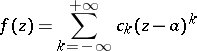(1)

with regular part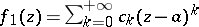and principal part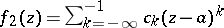. The behaviour of an analytic functionin a punctured neighbourhoodof an isolated singular point of single-valued character is determined, in principle, by the principal part of its Laurent series. If all the coefficients of the principal part are zero, then on setting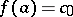one gets a single-valued analytic function in a full neighbourhood of. This case of practical absence of a singularity is also characterized by the fact thatis bounded in, or by the fact that the limit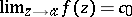,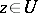, exists and is finite.

If among the coefficients of the principal part only finitely many are non-zero, and that with smallest index is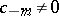, thenis a pole of order(cf. Pole (of a function)). A poleis also characterized by the fact that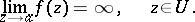Finally, if there are infinitely many non-zero coefficients in the principal part, thenis an essential singular point. In this case the following limit does not exist, neither finite nor infinite: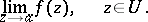For an isolated singular point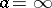at infinity of the element, a punctured neighbourhood has the form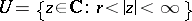, and the Laurent series is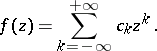Here the regular part is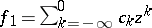and the principal part is. With these conditions, the above descriptions of the classification and criteria for the type of an isolated singular point carry over to the casewithout further change (see also Residue of an analytic function). It should be noted that the elements of different branches of the complete analytic functionat one and the same point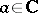may have singularities of completely-different types.

Holomorphic functionsof several complex variables,, cannot have isolated singular points. For, the singular points form infinite sets of singularities.

How to Cite This Entry:
Isolated singular point. Encyclopedia of Mathematics. URL: http://encyclopediaofmath.org/index.php?title=Isolated_singular_point&oldid=12648
This article was adapted from an original article by E.D. Solomentsev (originator), which appeared in Encyclopedia of Mathematics - ISBN 1402006098. See original article